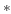Recorder
Subsections

## Recorder

This class allows you to record traces of any fields of any object during the simulation. The recorded traces of an Recorder with handle rec_idx can be obtained via

``` R=csim('get',rec_idx,'traces');
```
The ecxact form of R depends on the flag commonChannels (see below). Note that the traces returned always start at time \$t=0\$ and are recorded at an interval of dt.

In addition a recorder can also record spikes from spike emitting objects. Via a command like

``` csim('connect',rec_idx,neuron_idx,'spikes');
```
the Recorder with handle rec_idx is set up to record the spikes form the spike emitting objects with handles neuron_idx.

commonChannels=0 In this case the Matlab array R is a struct array with the only field channel which is in turn a struct array with the following fields:

• R.channel(j).idx : handle of the object from which field the data was recorded

• R.channel(j).spiking : binary flag (0/1) which determines if data should be interpreted as spike times or as an analog signal

• R.channel(j).dt : time discretization; for analog signals only

• R.channel(j).data : signal data : vector of the analog values or spike times.

• R.channel(j).fieldName : name of the recorded field

commonChannels=1 In this caseR has two fields (WARNING: no spikes are returned):

• R.data : A double array where R.data(j,s) is the s -th recorded value of the j -th field.

• R.info : A struct array where R.info(j).idx is the handle of the object from which the field R.info(j).fieldName is recorded.